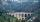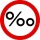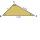5 km long road begins at an altitude 500 meters above sea level and ends at a altitude 521 ASL. How many permille road rises?

Result

p =  4.2

#### Solution:Leave us a comment of example and its solution (i.e. if it is still somewhat unclear...):Be the first to comment!## Next similar examples:

1. ClimbFor horizontal distance 4.2 km road rise by 6.3 m. Calculate the road pitch in ‰ (permille, parts per thousand).
2. Mountain railwayHeight difference between points A, B of railway line is 38.5 meters, their horizontal distance is 3.5 km. Determine average climb in permille up the track.
3. Plan scaleAt what scale is drawn plan of the building, where one side of the building is 45 meters long is on the plan expressed by a straight line 12 mm long.
4. ThomasThomas lives 400 meters away from Samko, Robo from Thomas also 400 m and Samko from Robo 500. Anton lives 300 meters away from Robo further as Samko. How far away lives Anton from Rob?
5. Scale 3Miriam room is 3.2 meters wide. It is draw by line segment length 6.4 cm on floor plan. In what scale it is plan of the room?
6. Obtuse angleWhich obtuse angle is creating clocks at 17:00?
7. AnglesThe outer angle of the triangle ABC at the vertex A is 114°12'. The outer angle at the vertex B is 139°18'. What size is the internal angle at the vertex C?Convert magnitude of the angle α = 9°39'15" to radians:
9. Down syndromeDown syndrome is one of the serious diseases caused by a gene mutation. Down syndrome occurs in approximately every 550-born child. Express the incidence of Down's syndrome in newborns at per mille.
10. Two municipalitiesThe horizontal distance between municipalities is 39 km. Average sinking is 7 per mille. What is the difference in height between these municipalities?
11. Center traverseIt is true that the middle traverse bisects the triangle?
12. Feet to milesA student runs 2640 feet. If the student runs an additional 7920 feet, how many total miles does the student run?
13. Internal and external anglesCalculate the remaining internal and external angles of a triangle, if you know the internal angle γ (gamma) = 34 degrees and one external angle is 78 degrees and 40 '. Determine what kind of triangle it is from the size of its angles.
14. Angles of a triangleIn the triangle ABC, the angle beta is 15° greater than the angle alpha. The remaining angle is 30° greater than the sum of the angles alpha and beta. Calculate the angles of a triangle.
15. Outer anglesThe outer angle of the triangle ABC at the A vertex is 71°40 ' outer angle at the vertx B is 136°50'. What size has the inner triangle angle at the vertex C?
16. Type of triangleHow do I find the triangle type if the angle ratio is 2:3:7 ?
17. Find the 9Find the missing angle in the triangle and then name triangle. Angles are: 95, 2x+15, x+3